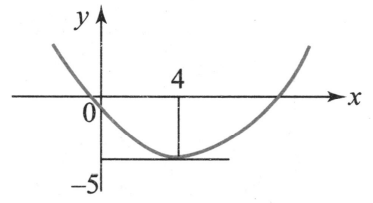Theory of expressions
Question

# The graph of the quadratic trinomial $\mathrm{y}={\mathrm{ax}}^{2}+\mathrm{bx}+\mathrm{c}$ has its vertex at (4, -5) and two x-intercepts, one positive and one negative. Which of the following holds good?

Moderate
Solution

##From figure,a > 0Also,

Get Instant Solutions